#### Truncated quadratic denoising - Penguin with hole (inpainting)

```Image size: 122 x 179, labels: 256
Data term: squared differences, except in hole where data costs = 0 for all labels
Smoothness term: squared differences, truncated at 200, lambda=25
```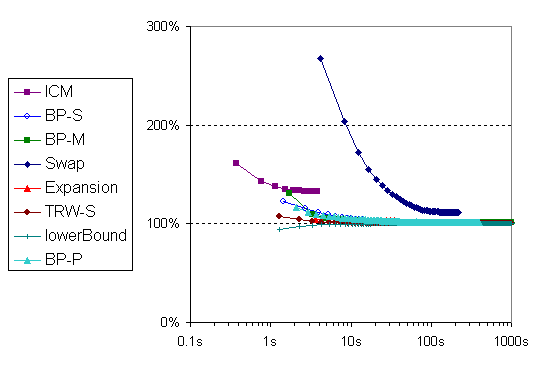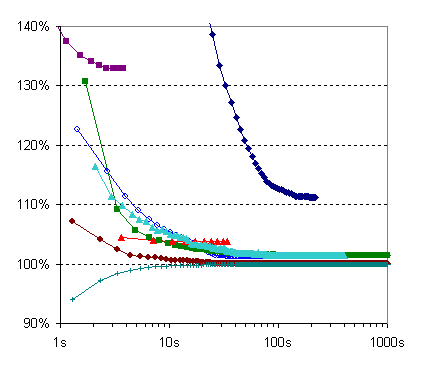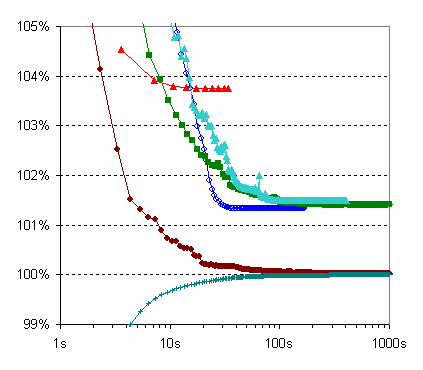Input image (noise contaminated), and the original image ("ground truth"):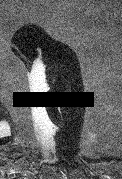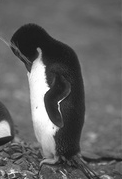(The black areas have data costs = 0 for all labels.)

All algorithms use the input intensities as start values.

Result images and their energies: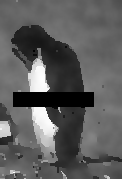max lower bound 15,344,406.9 ICM 20,393,445 (Ed= 9288220, Es= 11105225) BP-S 15,548,905 (Ed= 10984755, Es= 4564150) BP-M 15,560,021 (Ed= 10466496, Es= 5093525) Swap 17,076,141 (Ed= 10861541, Es= 6214600) Expansion 15,918,631 (Ed= 10810331, Es= 5108300) TRW-S 15,349,028 (Ed= 10807503, Es= 4541525) input image 162,606,700 (Ed= 0, Es=162606700) "ground truth" 50,311,716 (Ed= 8089591, Es= 42222125)